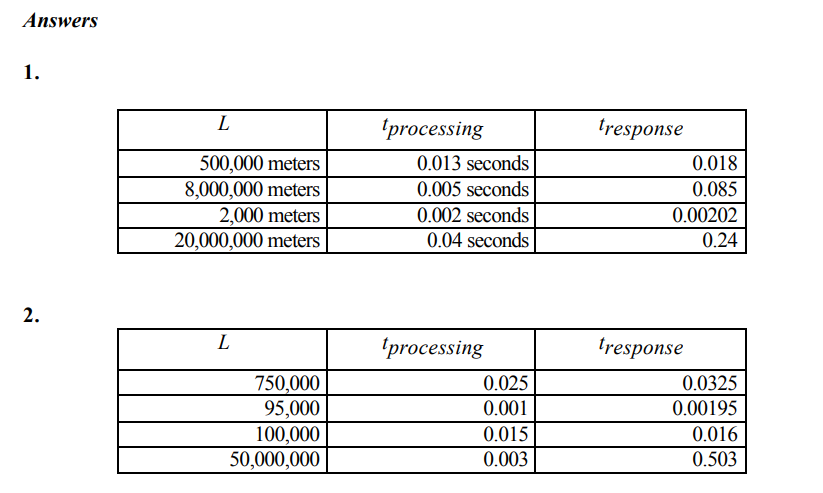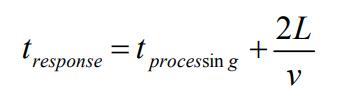Subject:
Mathematics

Topics: AlgebraExpressions and Equations
Common Core State Standard: A-CED.2, A-CED.4,
Knowledge and Skills:

· Can evaluate expressions by substituting values for variables
· Can simplify expressions using correct order of operations
· Can do basic operations on both sides of an equation in such a way as to preserve the equality

Lesson:### Response Time

If you send a signal over a fiber optic cable to another computer and get a response, the response time, tresponse, depends on three things:

a) the distance between you and the computer, L

b) the velocity of light traveling through the cable, v

c) the amount of time it takes the other computer to process your signal, tprocessing

The equation you would use to find the response time is this:Note that the velocity of light in fiber optic cable, v, is about 2/3 of the velocity of light in a vacuum, which makes it approximately equal to 200,000,000 meters/second.

1. Calculate tresponse for the given values of L and tprocessing:

 L tprocessing tresponse 500,000 meters 0.013 seconds 8,000,000 meters 0.005 seconds 2,000 meters 0.002 seconds 20,000,000 meters 0.04 seconds

2. Solve the equation to find the unknown value:

 L tprocessing tresponse 750,000 ? 0.0325 ? 0.001 0.00195 ? 0.015 0.016 50,000,000 ? 0.503